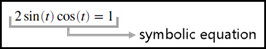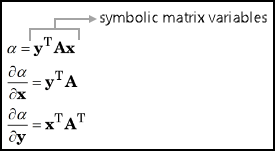Main Content

## Symbolic Objects to Represent Mathematical Objects

To solve mathematical problems with Symbolic Math Toolbox™, define symbolic objects to represent various mathematical objects. This example discusses the usage of the following symbolic objects:

• symbolic numbers

• symbolic scalar variables, functions, and expressions

• symbolic equations

• symbolic vectors and matrices

• symbolic matrix variables (since R2021a)

### Symbolic Number

Defining a number as a symbolic number instructs MATLAB® to treat the number as an exact form instead of using a numeric approximation. For example, use a symbolic number to represent the argument of an inverse trigonometric function $\theta ={\mathrm{sin}}^{-1}\left(1/\sqrt{2}\right)$.Create the symbolic number $\text{1/}\sqrt{2}$ using `sym`, and assign it to `a`.

`a = sym(1/sqrt(2))`
```a = 2^(1/2)/2```

Find the inverse sine of `a`. The result is the symbolic number `pi/4`.

`thetaSym = asin(a)`
```thetaSym = pi/4```

You can convert a symbolic number to variable-precision arithmetic by using `vpa`. The result is a decimal number with 32 significant digits.

`thetaVpa = vpa(thetaSym)`
```thetaVpa = 0.78539816339744830961566084581988```

To convert the symbolic number to a double-precision number, use `double`. For more information about whether to use numeric or symbolic arithmetic, see Choose Numeric or Symbolic Arithmetic.

`thetaDouble = double(thetaSym)`
```thetaDouble = 0.7854```

### Symbolic Scalar Variable, Function, and Expression

Defining variables, functions, and expressions as symbolic objects enables you to perform algebraic operations with those symbolic objects, including simplifying formulas and solving equations. For example, use a symbolic scalar variable, function, and expression to represent the quadratic function $f\left(x\right)={x}^{2}+x-2$. For brevity, a symbolic scalar variable is also called a symbolic variable.Create a symbolic scalar variable `x` using `syms`. You can also use `sym` to create a symbolic scalar variable. For more information about whether to use `syms` or `sym`, see Choose syms or sym Function. Define a symbolic expression `x^2 + x - 2` to represent the right side of the quadratic equation and assign it to `f(x)`. The identifier `f(x)` now refers to a symbolic function that represents the quadratic function.

```syms x f(x) = x^2 + x - 2```
```f(x) = x^2 + x -2```

You can then evaluate the quadratic function by providing its input argument inside the parentheses. For example, evaluate `f(2)`.

`fVal = f(2)`
```fVal = 4```

You can also solve the quadratic equation $f\left(x\right)=0$. Use `solve` to find the roots of the quadratic equation. `solve` returns the two solutions as a vector of two symbolic numbers.

`sols = solve(f)`
```sols = -2 1```

### Symbolic Equation

Defining a mathematical equation as a symbolic equation enables you to find the solution of the equation. For example, use a symbolic equation to solve the trigonometric problem $2\mathrm{sin}\left(t\right)\mathrm{cos}\left(t\right)=1$.Create a symbolic function `g(t)` using `syms`. Assign the symbolic expression `2*sin(t)*cos(t)` to `g(t)`.

```syms g(t) g(t) = 2*sin(t)*cos(t)```
```g(t) = 2*cos(t)*sin(t)```
To define the equation, use the `==` operator and assign the mathematical relation `g(t) == 1` to `eqn`. The identifier `eqn` is a symbolic equation that represents the trigonometric problem.
`eqn = g(t) == 1`
```eqn = 2*cos(t)*sin(t) == 1```

Use `solve` to find the solution of the trigonometric problem.

`sol = solve(eqn)`
```sol = pi/4```

### Symbolic Vector and Matrix

Use a symbolic vector and matrix to represent and solve a system of linear equations.

`$\begin{array}{c}x+2y=u\\ 4x+5y=v\end{array}$`

You can represent the system of equations as a vector of two symbolic equations. You can also represent the system of equations as a matrix problem involving a matrix of symbolic numbers and a vector of symbolic variables. For brevity, any vector of symbolic objects is called a symbolic vector and any matrix of symbolic objects is called a symbolic matrix.Create two symbolic equations `eq1` and `eq2`. Combine the two equations into a symbolic vector.

```syms u v x y eq1 = x + 2*y == u; eq2 = 4*x + 5*y == v; eqns = [eq1, eq2]```
```eqns = [x + 2*y == u, 4*x + 5*y == v]```

Use `solve` to find the solutions of the system of equations represented by `eqns`. `solve` returns a structure `S` with fields named after each of the variables in the equations. You can access the solutions using dot notation, as `S.x` and `S.y`.

```S = solve(eqns); S.x```
```ans = (2*v)/3 - (5*u)/3```
`S.y`
```ans = (4*u)/3 - v/3```

Another way to solve the system of linear equations is to convert it to matrix form. Use `equationsToMatrix` to convert the system of equations to matrix form and assign the output to `A` and `b`. Here, `A` is a symbolic matrix and `b` is a symbolic vector. Solve the matrix problem by using the matrix division \ operator.

`[A,b] = equationsToMatrix(eqns,x,y)`
```A = [1, 2] [4, 5] b = u v```
`sols = A\b`
```sols = (2*v)/3 - (5*u)/3 (4*u)/3 - v/3```

### Symbolic Matrix Variables

Since R2021a

Use symbolic matrix variables to evaluate differentials with respect to vectors.

`$\begin{array}{l}\alpha ={y}^{\text{T}}Ax\\ \frac{\partial \alpha }{\partial x}={y}^{\text{T}}A\\ \frac{\partial \alpha }{\partial y}={x}^{\text{T}}{A}^{\text{T}}\end{array}$`

Symbolic matrix variables represent matrices, vectors, and scalars as atomic symbols. Symbolic matrix variables offer a concise display in typeset and show mathematical formulas with more clarity. You can take vector-based expressions from textbooks and enter them in Symbolic Math Toolbox.Create three symbolic matrix variables `x`, `y`, and `A` using the `syms` command with the `matrix` argument. Nonscalar symbolic matrix variables are displayed as bold characters in the Command Window and in the Live Editor.

```syms x [4 1] matrix syms y [3 1] matrix syms A [3 4] matrix x y A```
```x = x y = y A = A```
Define `alpha`. Find the differential of `alpha` with respect to the vectors x and y that are represented by the symbolic matrix variables `x` and `y`.
`alpha = y.'*A*x`
```alpha = y.'*A*x```
`diff(alpha,x)`
```ans = y.'*A```
`diff(alpha,y)`
```alpha = x.'*A.'```

### Comparisons of Symbolic Objects

This table compares various symbolic objects that are available in Symbolic Math Toolbox.

Symbolic ObjectsExamples of MATLAB CommandsSize of Symbolic ObjectsData Type
symbolic number
```a = 1/sqrt(sym(2)) theta = asin(a)```
```a = 2^(1/2)/2 theta = pi/4```
`1`-by-`1``sym`
symbolic scalar variable
`syms x y u v`
`1`-by-`1``sym`
symbolic function
```syms x f(x) = x^2 + x - 2 syms g(t) g(t) = 2*sin(t)*cos(t)```
```f(x) = x^2 + x - 2 g(t) = 2*cos(t)*sin(t)```
`1`-by-`1``symfun`
symbolic equation
```syms u v x y eq1 = x + 2*y == u eq2 = 4*x + 5*y == v```
```eq1 = x + 2*y == u eq2 = 4*x + 5*y == v```
`1`-by-`1``sym`
symbolic expression
```syms x expr = x^2 + x - 2 expr2 = 2*sin(x)*cos(x)```
```expr = x^2 + x - 2 expr2 = 2*cos(x)*sin(x)```
`1`-by-`1``sym`
symbolic vector
```syms u v b = [u v]```
```b = [u, v]```
`1`-by-`n` or `m`-by-`1``sym`
symbolic matrix
```syms A x y A = [x y; x*y y^2]```
```A = [ x, y] [x*y, y^2]```
`m`-by-`n``sym`
symbolic multidimensional array
```syms A [2 1 2] A```
```A(:,:,1) = A1_1 A2_1 A(:,:,2) = A1_2 A2_2```
`sz1`-by-`sz2`-...-`szn``sym`
symbolic matrix variable (since R2021a)
```syms A B [2 3] matrix A B```
```A B```
`m`-by-`n``symmatrix`

## Support

#### Mathematical Modeling with Symbolic Math Toolbox

Get examples and videos